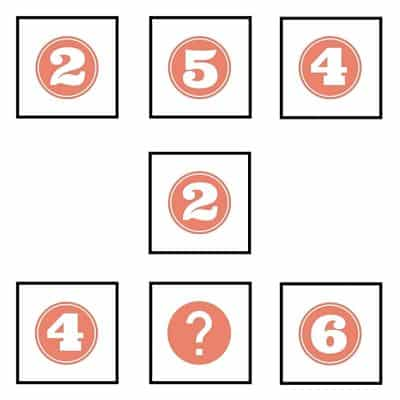#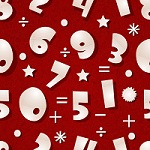Logic Number Puzzles

We're a huge fan of logic puzzles here at LaffGaff.

So if you are too, here's a great selection of number puzzles for you to give your brain a workout.

How many will you get right?

Which number completes the number in the sequence below?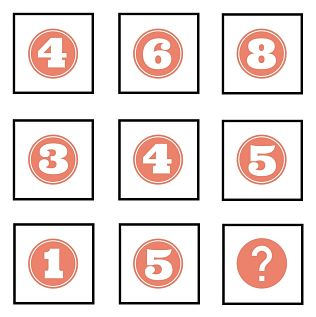The missing number is 9.

For each row, the middle number is the average of the left number and the right number. For example, 4 + 8 / 2 = 6.

Which number should be placed in the center of the third triangle below?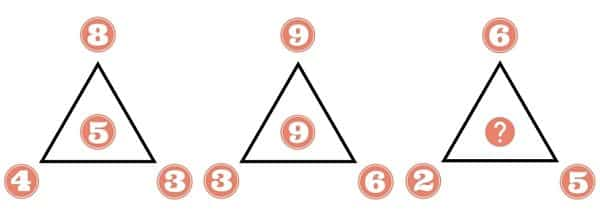The missing number is 8.

The center number of each triangle is found by dividing the top number by the bottom left number and adding the result to the bottom right number.

Which number goes in the middle of the square to complete the sequence?The missing number is 3.

The sum of the numbers in each row and column is 12.

Which number completes the sequence below?The missing number is 13.

For each column, the next number down is equal to twice the number above it minus 1.

Which number is missing from the lower left circle?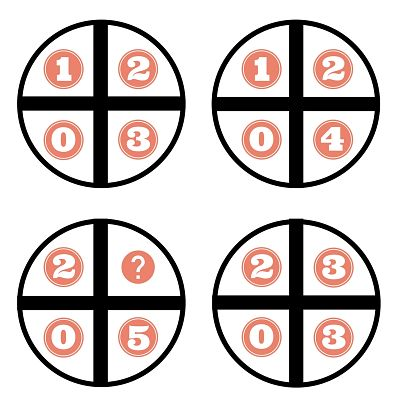The missing number is 2.

Starting with the top left circle, and moving in a clockwise direction, the numbers in each circle add up to 6, 7, 8 and then 9.

Which number is missing from the square below?